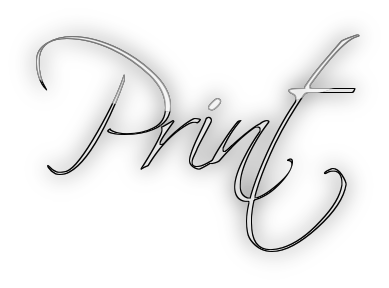# Print Function in R - Cat Function in RSimply enter the variable name or expression at the command prompt, R will print its value. Use the print function for generic printing of any object. Use the cat function for producing custom formatted output. Here is the demonstration.

``pi``

## Print Function

Another way to print pi using Print function as shown below

``print(pi)``

This is useful because you can always view your data: just print it. You needn’t write special printing logic, even for complicated data structures.

The print function has a significant limitation, however: it prints only one object at a time. Trying to print multiple items gives this mind-numbing error message:

Error in print.default("The zero occurs at", pi, "radians.") :
invalid 'quote' argument
Calls: print -> print.default
Execution halted

``print("The zero occurs at", pi, "radians.")``

## Cat FunctionThe cat function is an alternative to print that lets you combine multiple items into a continuous output:

``cat("The zero occurs at", 2*pi, "radians.", "\n")``

Using cat gives you more control over your output, which makes it especially useful in R scripts. A serious limitation, however, is that it cannot print compound data structures such as matrices and lists.

Error in cat(list(...), file, sep, fill, labels, append) :
argument 1 (type 'list') cannot be handled by 'cat'
Execution halted

``cat(list("a","b","c"))``Scroll to Top